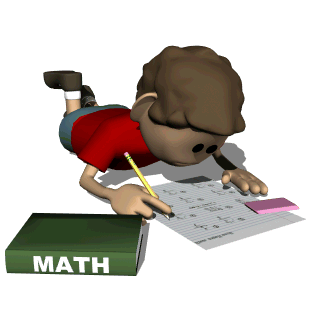UPDATED!!  Most apps are free but if they are not, I did include a price.

1. My Math App- Students can practice addition, subtraction, multiplication and division facts with randomly generated flashcards. K-5

2. Math Puppy- Bingo and math challenges for students. Level of difficulty can be adjusted but subtraction is the only free operation. K-2

3. Animal Math Games for Kids- Students can count to 10, do patterns and sequences and addition to 5. K

4. Animal Math for 1st Grade- Students can practice greater, less than skills, number sentences and subtraction. 1st grade

5. 123 Genius First Numbers and Counting Game for Kids- Students can practice counting, writing and matching numbers to 10. K

6. Counting 123-Learn to Count- Students learn count 1-10 with 4 fun mini games. K

7. My Math Helper- Students can practice adding and subtracting as well as writing numbers. Pictures are also included to help with counting. K-1

8. Math Fish Tank- Students earn tokens by solving addition, subtraction, multiplication and division problems to acquire items for their fish tank. High interest!! K- 3 \$0.99

9. Motion Math Zoom- Students can practice number order. K-1

10. Addition and Multiplication Number Bubbles- choose between addition and multiplication games. Three different modes to choose from. 2-3

11. Mathmateer- Students can practice a variety of place value concepts. Fun rocket launches makes this app very appealing to students. High Interest!!!  1-5 \$1.99

12. Arithmetic Invaders Express- Students can practice number order and addition, subtraction and multiplication facts by shooting at space invaders to defend the planet. High Interest!!!  K-3

13. AB Math-Students can practice addition and multiplication skills. 1-5

14. Butterfly Math-Students can practice counting skills, as well as addition facts with 3 different levels. K-3

15. Math Kids Free-Students can practice addition, subtraction, and counting. There is a set up button to control which skill to practice. K-1

16. Rounding Numbers-Students can practice rounding whole numbers to the tens and hundreds, the number of questions as well as a timer can also be set up. 2-5

17. Kindergarten Math-Students can practice addition, subtraction and comparing numbers. K-2

18. Splash Math Grade 1 & 2-Students can practice place value, as well as practice addition and subtraction facts. K-2

19. First Grade Learning Games- Students can practice patterns and ordering. K-1

20. Math Zombies- Students can practice addition, subtraction, multiplication and division while avoiding zombies. There is easy, medium and hard level. Addition and subtraction is single, double and triple digit. High Interest!!! Grades 1-5

21. Math vs. Undead math game- Students can practice basic math operations, such as addition, subtraction, multiplication and division. K-5 High Interest!!

22. Monster Math-Games for Kids- Students can practice basic geometry, addition, subtraction, multiplication and division. K-5

23. Math Evolve- Students practice addition, subtraction, multiplication and division skills while shooting at aliens and flying in space. There are 3 levels of difficulty, which include regrouping with addition and subtraction.  Students can also chose level of game difficulty from beginner to expert. High interest! 1-5 \$1.99

24. Math Express Free-Students practice addition facts to 10. K-2

25. Love to Count- Students practice number sense. Cute pirate theme. High interest.  K-2 \$3.99

26. Count 1,2,3- A basic counting app where students touch a number and it is read to them as an amount is shown. K

27. Math Island 1st Class- Students can practice addition, subtraction, multiplication and division facts from 5 different levels. 1-5

28. Subtraction Free- Students can practice subtraction skills. The number of digits can be 1-2 and regrouping problems are also included. 2-5

29.  Geoboards- This app is a virtual geoboard where students can practice making shapes. K-5

30. Math Bingo- Students can practice addition, subtraction, multiplication, and division facts, with 3 levels of difficulty. 1-5

31. Sushi Monster- (from Scholastic) Students can practice either multiplication or addition by finding number pairs that equals the given number. Level of difficulty increases as student plays increasing to hundreds in both addition and multiplication. High interest! 2-5

32. Arithmetic Whiz- Students practice addition, subtraction, multiplication and division skills. Students score and time is displayed at the end. 1-5

33. Counting Money- Students choose realistic looking coins to make a given amount.  In the setting there are three levels of difficulty ranging from cents to dollars and a practice and quiz mode.

34. Counting Coins- Students can count pennies, nickels, dimes and quarters. iphone only

35. Amazing Coin-Students play a variety of games including identification of coin and value 1-3